# Solving Systems of Trigonometric Equations

Instructor
Michael Quist

Michael has taught college-level mathematics and sociology; high school math, history, science, and speech/drama; and has a doctorate in education.

Expert Contributor
Robert Ferdinand

Robert Ferdinand has taught university-level mathematics, statistics and computer science from freshmen to senior level. Robert has a PhD in Applied Mathematics.

Trig equations get crazy enough, but when you have systems of them, it can get a little overwhelming. In this lesson, we'll explore some easy tactics for make solving systems of trig equations much easier. Updated: 06/02/2020

## What are Systems of Trigonometric Equations?

Systems of trigonometric equations can easily begin to look like some language from Mars (or maybe Brooklyn), but there are a few tricks of the trade that make solving systems of these arcane creatures a lot easier.

Trigonometric expressions are mathematical expressions that involve the ratios of the sides of a right triangle, which allow you to work with rotational angles. A trigonometric equation is an algebraic-style equation that includes trigonometric expressions.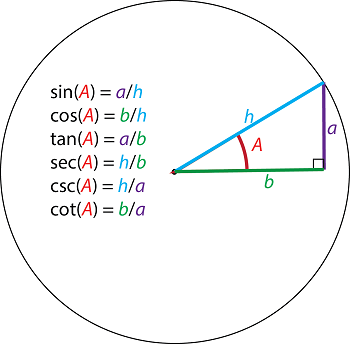Any time you see a trig identity, such as sin(x), show up in an equation, you know that your solution will be an angle, a measure of rotation. For example, if you are given the equation sin(x) + cos(y) = 1, then you know that two angles (x and y) will be involved. Since angles are rotational (like a bicycle wheel), they repeat at various positions around the axle. For example, the sine is .5 when the angle is 30°, 150°, and many other positions. You always define the range of the answers you want when you're working with trigonometric equations, such as the rotational angles between 0° and 360°.

## Solving Systems of Equations

Systems of equations (a group of equations that are all related) can usually be solved (or be shown to have no solutions), so long as you have at least one equation for each variable that shows up. Solving any system of equations usually involves a mix of substitution and combination methods. Remember, substitution means you grab a definition for one of the variables (usually by solving one of the equations for that variable in terms of the others), and then plug it into the other equation (or one of the other equations). This would be one way to simplify the following system:

Equation 1: x + y = 1

Equation 2: x - y = -3

Solving the first equation for 'x', you have a definition for x:

x + y = 1

x = -y + 1 (Subtract 'y' from both sides)

You can use this definition for 'x' in Equation 2:

x - y = -3

(-y + 1) - y = -3 (Substitute -y + 1 for x)

-2y = -4 (Subtract 1 from both sides)

y = 2 (Divide both sides by -2)

Combination involves adding two or more equations together in the hope of hiding one of the variables so you can work with the other (or others). Since both sides of each equation are equal, adding them to another equation will not affect its equality. For example, you can use combination on Equations 1 and 2, instead of substitution.

Equation 1: x + y = 1

Equation 2: x - y = -3

When the two equations are added together, the y variable disappears.

2x = -2 (Simplify)

x = -1 (Divide both sides by 2)

## Solving a System of Trigonometric Equations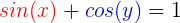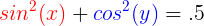Now let's apply our techniques to this system of trigonometric equations. First, let's start by temporarily getting rid of the trig expressions until we're ready for them. We'll rename the sin(x) expression as a, and the cos(y) as b. That will make our algebraic work a lot simpler. When we're ready to do the trig part, we'll put the original expressions back.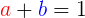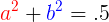Notice, we just squared the a and b for the second equation, to match what was being done to the original function. Using the substitution principle we'll get rid of one of the variables. By subtracting b from both sides of the first equation, we can define a as '1 - b':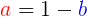Now we'll plug that value for a into the 2nd equation: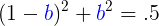Multiplying out the binomial, you have the makings of a quadratic equation: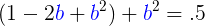Let's re-arrange it to make it look more like the quadratic that it is: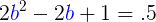Subtracting .5 from both sides, we have standard form for a quadratic equation.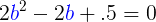Dividing that equation by 2, we land on a simpler quadratic: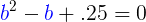To unlock this lesson you must be a Study.com Member.

## Systems of Trigonometric Equations: Practice Problems

### Key Terms

• Trigonometric Equations: Contain trigonometric functions of unknowns.
• System of Equations: More than one equation in more than one unknown.

• Paper
• Pencil
• Calculator

### Practice Problems (Show all Your Work) -

(a) Find angle pairs (x, y) in radians, in the interval [0, 2pi], that solve the following system of equations:

cos (x) + cos (y) = 1 [Equation 1]

cos (x) - cos (y) = 0 [Equation 2]

(b) Find angle pairs (x, y) in radians, in the interval [0, 2pi], that solve the following system of equations:

tan (x) - tan (y) = 4 [Equation 1]

tan (x) = 2 - tan (y) [Equation 2]

(a) Use the method of elimination as follows:

Step 1: Add the two equations.

2 cos (x) = 1.

Step 2: Divide both sides by 2.

cos (x) = 1/2.

Step 3: Take inverse cosine of both sides.

Step 4: Substitute the values of x in Equation (2).

cos (y) = 1/2.

Step 5: Take inverse cosine once again to yield.

Hence, the solution set is the ordered pairs (x, y) = (pi/3, pi/3), (pi/3, 5pi/3), (5pi/3, pi/3), (5pi/3, 5pi/3).

(b) Use the method of substitution as follows:

Step 1: Substitute tan (x) = 2 - tan (y) from Equation (2) into Equation (1) to arrive at

2 - 2 tan (y) = 4

Step 2: Subtract 2 from both sides.

-2 tan (y) = 2

Step 3: Divide both sides by -2.

tan (y) = -1

Step 4: Take inverse tangent of both sides.

y = 3pi/4 and 7pi/4 (radians)

Step 5: Substitute y in Equation (1).

tan (x) + 1 = 4 or tan (x) = 3

Step 6: Using a calculator we get x = 1.25 and x = 4.39 radians.

Hence, the solution set is the ordered pairs (x, y) = (1.25, 3pi/4), (1.25, 7pi/4), (4.39, 3pi/4), (4.39, 7pi/4).

### Register to view this lesson

Are you a student or a teacher?